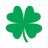# Linear Regression Slope Angle Plot##### Member
I slightly modified this linear regression slop study to include an angle label. I'm looking to further improve it by adding another angle label that changes the angel value as I hover my pointer over the slope line. Can someone please help:

Code:
``````declare lower;

input price = close;
input length = 14;
input displace = 0;
def avg = ExpAverage(close, length);
def height = avg - avg[length];
def Angle_deg = ATan(height/length) * 180 / Double.Pi;
addLabel(1, "Angle Deg " + Angle_deg, Color.WHITE);

Assert(length > 1, "'length' must be greater than one: " + length);

plot LRS = 6 * ( wma(price, length) -  Average(price, length) ) / (length - 1);
plot ZeroLine = 0;

LRS.setDefaultColor(getColor(8));
ZeroLine.setDefaultColor(getColor(5));``````

•ezrollin and markosLinear Regression Trading Strategy for ThinkorSwim Strategies 14